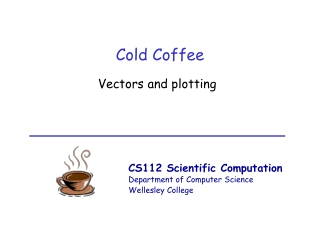DownloadDownload PresentationCold Coffee

# Cold Coffee

Télécharger la présentation## Cold Coffee

- - - - - - - - - - - - - - - - - - - - - - - - - - - E N D - - - - - - - - - - - - - - - - - - - - - - - - - - -
##### Presentation Transcript

1. Cold Coffee Vectors and plotting

2. Cold, funny-tasting coffee Time(min) Temperature (°C) 0 93.5 1 90.6 2 87.7 3:30 83.9 7 76.6 10 71.3 18 61.5 26 65.8 34 49.2 52 41.9 113 29.1 166 25.5 191 25.0 Vectors

3. Vectors • A vector is an ordered collection of numbers times = [0 1 2 3.5 7] temps = [93.5 90.6 87.7 83.9 76.6] times 0 1 2 3.5 7 temps 93.5 90.6 87.7 83.9 76.6 Vectors

4. Plotting data: Round 1 • Create a 2D graph with the plot function times = [0 1 2 3.5 7] temps = [93.5 90.6 87.7 83.9 76.6] plot(times, temps) vector of x coordinates vector of y coordinates Vectors

5. Computations on collections of data • Perform the same arithmetic operation on each element of vector >>times = [0 1 2 3.5 7] >> times = 60 * times times 0 1 2 3.5 7 times 60 0 120 210 420 Vectors

6. Time-out exercise • Write an assignment statement to convert temperature from Celcius to Fahrenheit* >> temps = [93.5 90.6 87.7 83.9 76.6] >> ... temps 93.5 90.6 87.7 83.9 76.6 temps 200.3 195.08 189.86 183.02 169.88 * Hint: F = 32 + (9/5)C Vectors

7. More vector magic • Perform element-by-element computations on two vectors, e.g. average the two data samples* >> sample1 = [3.2 1.1 4.7 2.1 6.2] >> sample2 = [3.0 1.2 4.4 2.4 6.1] sample1 3.2 1.1 4.7 2.1 6.2 3.0 1.2 4.4 2.4 6.1 sample2 * Hint: How do you calculate the average of two single numbers sample1 and sample2? Vectors

8. The envelope please • Element-by-element average: >> sample1 = [3.2 1.1 4.7 2.1 6.2] >> sample2 = [3.0 1.2 4.4 2.4 6.1] >> average = (sample1 + sample2) / 2 sample1 3.2 1.1 4.7 2.1 6.2 sample2 3.0 1.2 4.4 2.4 6.1 average 3.1 1.15 4.55 2.25 6.15 Vectors

9. Let’s try that one again • Perform element-by-element computations on two vectors, e.g. multiply corresponding values in the two data samples* >> sample1 = [3.2 1.1 4.7 2.1 6.2] >> sample2 = [3.0 1.2 4.4 2.4 6.1] sample1 3.2 1.1 4.7 2.1 6.2 sample2 3.0 1.2 4.4 2.4 6.1 * oops… Vectors

10. Still more magic • Compute a single value from the contents of a vector >> sample1 = [3.2 1.1 4.7 2.1 6.2] >> totalSum = sum(sample1) >> totalProd = prod(sample1) >> sampleSize = length(sample1) >> minValue = min(sample1) >> maxvalue = max(sample1) >> avgValue = mean(sample1) sample1 3.2 1.1 4.7 2.1 6.2 Vectors

11. Creating sequences • Often you will want to create a sequence of evenly spaced numbers* • Use colon notation >> nums = 1:2:9 end start step nums 1 3 5 7 9 * Trust me, you really will want to do this someday! Vectors

12. Creating evenly spaced sequences • Use the linspace function: linspace(start, end, num) to create a vector of num evenly spaced numbers from startto end >> nums = linspace(0, 10, 5) nums 0.0 2.5 5.0 7.5 10.0 * Write an assignment statement that stores a sequence of 5000 evenly spaced numbers from 1 to 1000 Vectors

13. Plotting data: Round 2 • Adjust the appearance of lines and markers with a third input to the plot function: plot(times,temps,‘g--*’); • Third input is a string of 1 to 4 characters in single quotes: color:b g r c m y k w marker: . o x + * s d v ^ < > p h linestyle: - : -. -- • Add text labels: xlabel(‘Time, minutes’) ylabel(‘Temperature, Celcius’) title(‘Cooling a Cup of Coffee’) Vectors

14. Newton’s Law of Cooling • An object’s rate of cooling depends on the difference between object’s temperature and ambient temperature • Temperature decreases exponentially over time • According to Newton: T(t) = Tenv + (T(0) - Tenv)e-kt where T(t) is object temperature at time t T(0) is initial temperature of object Tenvis environment temperature kdepends on material (for coffee k ~ 0.0426) Vectors

15. Coffee break % cooling.m % compares the direct measurement of cooling of coffee with % the cooling rate predicted by Newton’s Law of Cooling timeData = [0 1 2 3.5 7 10 18 34 52 113 166 191]; tempData = [93.5 90.6 87.7 83.9 76.6 71.3 61.5 54.8 ... 49.2 41.0 29.1 25.5 25.0]; plot(timeData, tempData, ‘b-*’) xlabel(‘Time, minutes’) ylabel(‘Temperature, degrees Celcius’) title(‘Cooling a Cup of Coffee’) timeSamples = 0:5:200; tempLaw = 24.5 + 69 * exp(-0.0426 * timeSamples); hold on plot(timeSamples, tempLaw, ‘g--o’) hold off Vectors

16. Ta Da! Vectors

17. Exercise: Center of Mass % coordinates and masses of particles xcoords = [1 7 6 8 3 5 9 2 8 6 2 4 1]; ycoords = [8 3 7 5 9 1 3 6 8 8 2 3 4]; masses = [11 8 10 4 5 11 6 7 12 8 9 6 5]; % calculate the center of mass of the particles xcenter = ycenter = % print center of mass coordinates using disp Vectors